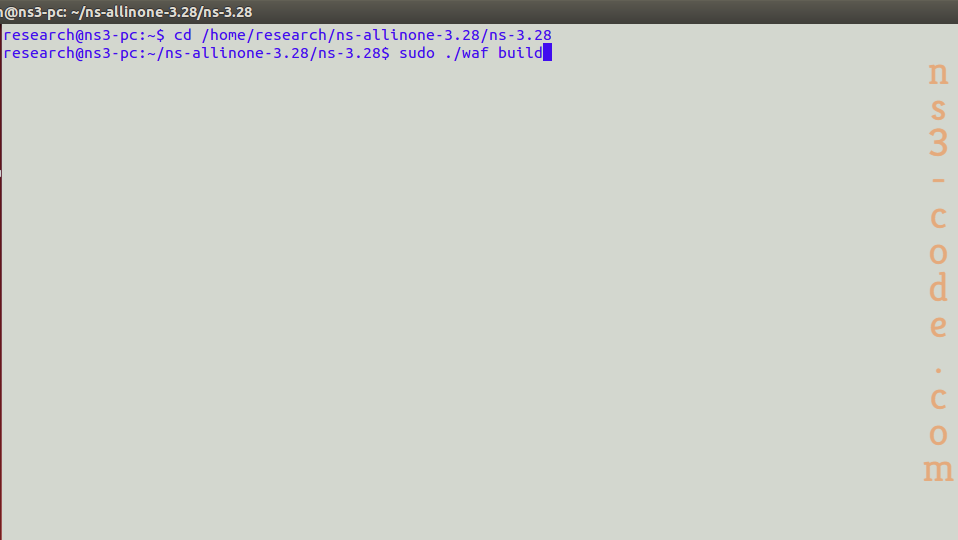## What Can I Do With Ns3

In fact, network simulator 3 is mainly deployed in the connection modules for the creation of wireless, point to point and various connections among nodes.

For instance, we have highlighted some implementation codes based on network simulator in the following.

• Point to point configuration
``` Ptr n0 = CreateObject (); Ptr net0 = CreateObject (); n0->AddDevice (net0); Ptr q = CreateObject (); net0->SetQueue (q); PointerValue ptr; net0->GetAttribute ("TxQueue", ptr); Ptr txQueue = ptr.Get (); Ptr dtq = txQueue->GetObject (); ```
• Wireless configurations
``` WimaxHelper wimax; channel = CreateObject (); channel->SetPropagationModel (SimpleOfdmWimaxChannel::COST231_PROPAGATION); ssDevs = wimax.Install (ssNodes, WimaxHelper::DEVICE_TYPE_SUBSCRIBER_STATION, WimaxHelper::SIMPLE_PHY_TYPE_OFDM, channel, scheduler); Ptr dev = wimax.Install (bsNodes.Get (0), WimaxHelper::DEVICE_TYPE_BASE_STATION, WimaxHelper::SIMPLE_PHY_TYPE_OFDM, channel, scheduler); ```

Following that, we have highlighted the commands that are implemented to build the simulation code in ns3.

``` cd /home/research/ns-allinone-3.28/ns-3.28 sudo ./waf build ```